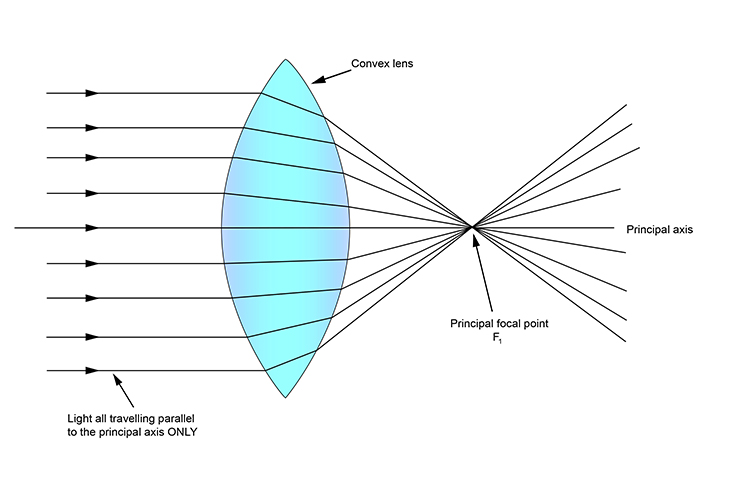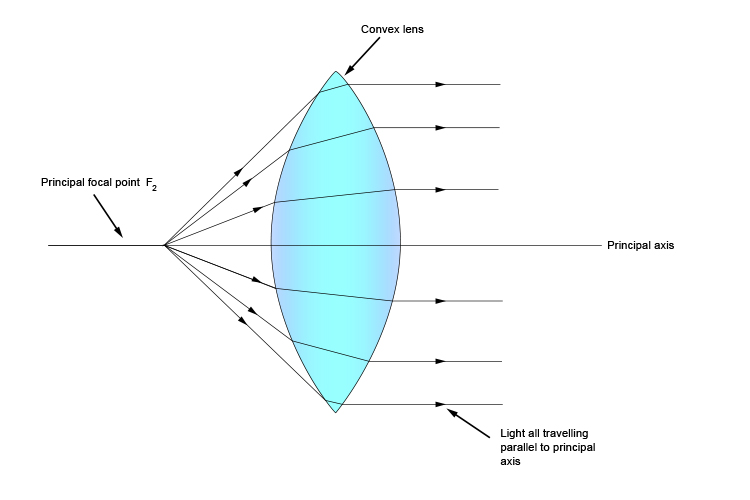# Principal focal point (two of them)

The most important characteristic of a lens is its principal focal point.

We know the term principal axis and this gives us a clue that we are talking about a point on the principal axis. However, the word "focal" is confusing. The term "convergence point" is perhaps a better description of the principal focal point. This convergence point only applies to light that is passing parallel to the principal axis – see below:When parallel rays of light pass through a convex lens, the refracted rays converge at one point called the principal focal point.

This does not mean it focuses an image at this point, it only means it's a point where parallel rays will all pass through. Call it the principal focus (convergence) point.

However, a convex lens has two spherical surfaces and therefore it has two principal foci or two focal points.

The second principal focus is that point on the principal axis from which all incoming rays, after refraction, become parallel.